# Lee Plath

•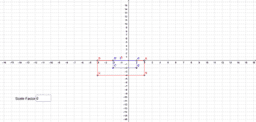### Find the scale factor

Activity

Lee Plath

•### Solve equations with variables on both sides.

Activity

Lee Plath

•### Solving two-step equations.

Activity

Lee Plath

•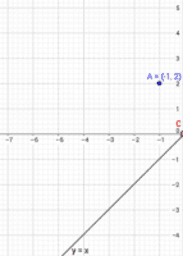### Explore reflections

Activity

Lee Plath

•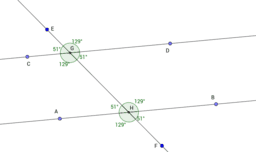### Parallel lines cut by a transversal.

Activity

Lee Plath

•### Parallel lines cut by a transversal

Activity

Lee Plath

•### Parallel lines cut by a transversal

Activity

Lee Plath

•### Solve the equations

Activity

Lee Plath

•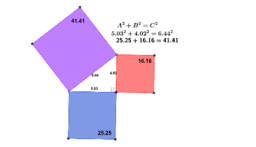### Pythagorean theorem

Activity

Lee Plath

•### Slope intercept

Activity

Lee Plath

•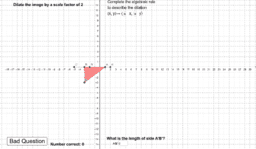### Algebraic dilations

Activity

Lee Plath

•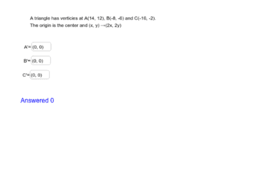### algebra dilations

Activity

Lee Plath

•### Find the scale factor of the dilation

Activity

Lee Plath

•### Slope intecept form.

Activity

Lee Plath

•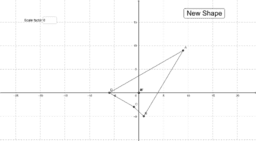### Adjusting the scale factor of a dilation

Activity

Lee Plath

•### Algebra dilations

Activity

Lee Plath

•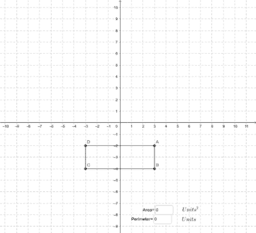### Finding area and perimeter

Activity

Lee Plath

•### Exploring Chords

Activity

Lee Plath

•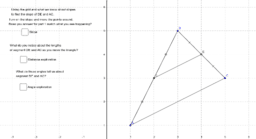### Midpoints of triangles

Activity

Lee Plath

•Activity

Lee Plath